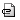## Anthracene †

This tutorial explains how to obtain the maximally localized Wannier function of an anthracene crystal. See Japanese version.

### Relaxation †

First of all, optimize the atomic positions as well as the cell parameters.

### SCF calculation †

A single point (SCF) calculation is performed by using the optimized structure (using the input filec28h20.scf.in).

### Non-SCF calculation †

To prepare for the calculation of the max. loc. Wannier function, non-SCF calculation using a k-point set generated by using utility/kmesh.pl. To generate, for instance, a 4 x 4 x 4 k-point set, execute

`kmesh.pl 4 4 4`

and use the generated k-points to create the input file for the non-SCF calculation (c28h20.nscf.in)

### Post-processing †

#### wannier90.x -pp †

After the non-SCF calculation, *.win to be generated first and one uses the following commands. Let us namec28h20.win and then execute

`wannier90.x -pp c28h20`

#### pw2wannier90.x †

Then execute pw2wannier90.x as other commands in the QE suite such as pw.x using the input file (e.g.,c28h20.pw2wan). Note the same number of cores should be used as the SCF/Non-SCF calculation if wf_collect=.false.

#### wannier90.x †

Finally execute wannier90.x

`wannier90.x c28h20`

Note Wannier90 (<3) is not parallelized and it is not necessary to use mpirun nor the same number of cores for SCF and non-SCF calculations.

Last-modified: 2019-12-11 (水) 23:48:10 (322d)